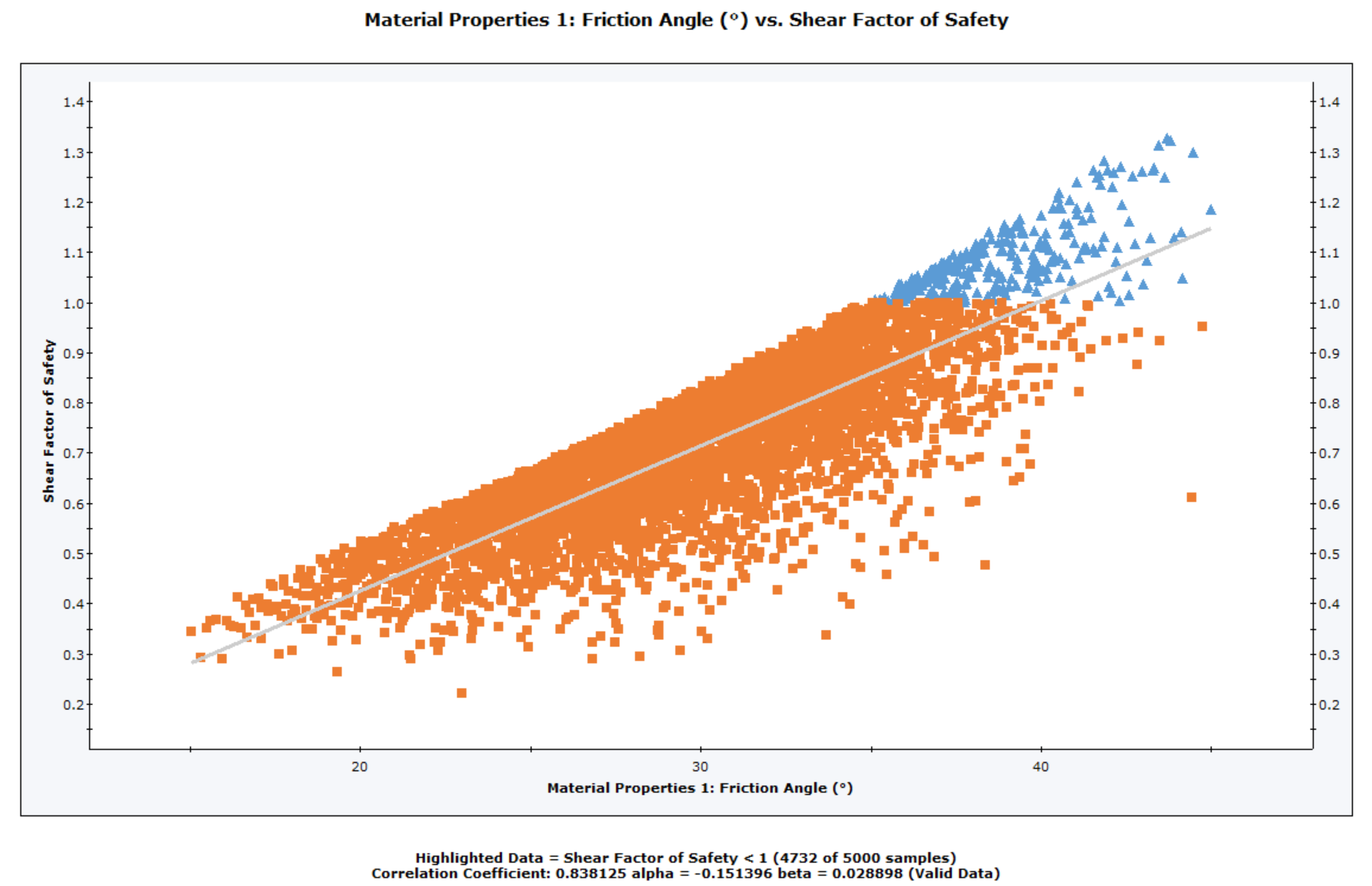The Rocscience International Conference 2021 Proceedings are now available. Read Now

# Scatter Plot

To create a Scatter Plot after a Probabilistic Analysis:

1. Select Statistics > Plot Scatter or the "Plot Scatter"toolbar button.
2. Select the Failure Mode (e.g. Shear, Elastic Buckling, Arch Snap Thru, or Compression, whichever is applicable).
3. Select the data you would like to plot on the X-axis and Y-axis.
4. Highlight Data - if you want to highlight data with safety factor less than a particular number, select the Highlight Data check box and enter a value of safety factor (default = 1).
5. Select OK.

The Scatter Plot will be generated. At the bottom of the Scatter Plot, you will see a listing of the Correlation Coefficient, alpha and beta.

Scatter Plot with linear regression best fit displayed## Correlation Coefficient

The Correlation Coefficient indicates the degree of correlation between the two variables plotted. The Correlation Coefficient can vary between -1 and 1 where numbers close to zero indicate a poor correlation, and numbers close to 1 or -1 indicate a good correlation. Note that a negative correlation coefficient simply means that the slope of the best fit linear regression line is negative.

## Alpha and Beta

Alpha and beta represent the y-intercept and slope, respectively, of the best fit linear regression line to the scatter plot data. The linear regression line can be displayed on the plot with the Regression Line option in the right-click menu.

Several options are available in the right-click menu for Scatter Plots, including: